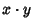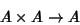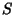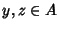## Zero Divisor

A Nonzero elementof a Ring for which, whereis some other Nonzero element and the vector multiplicationis assumed to be Bilinear. A Ring with no zero divisors is known as an Integral Domain. Letdenote an-algebra, so thatis a Vector Space overandNow definewhere.is said to be-Associative if there exists an-dimensional Subspaceofsuch thatfor alland.is said to be Tame ifis a finite union of Subspaces of.

References

Finch, S. Zero Structures in Real Algebras.'' http://www.mathsoft.com/asolve/zerodiv/zerodiv.html.